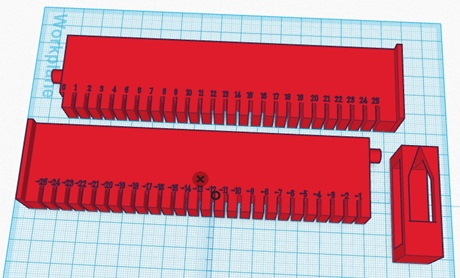# Negative and Positive Number LineLearning about positive and negative numbers is a concept that can fall prey to memorization of rules without understanding rationale. Negative values can easily become abstract and conceptual; unrelated to a concrete idea. Until the moment of learning negative numbers, students learn that all numbers represent solid quantities. Even when understanding fractions and decimals, the quantities can be represented by shaded areas, blocks, etc. But the question arose as to what manipulative exists to represent negative quantities.

While researching how to exemplify the concept of positive and negative numbers to a student, other ideas included thermometers and elevators with subterraneous floors. These examples were beneficial in illustrating real life examples of negative values. Their functioning also involved number lines to show where their value was at any given moment.

Number lines are beneficial as they teach numbers in relationship to each other as opposed to single units (Van de Walle, 2014, p.106). A number line that extends from negative twenty-five through twenty five, would offer students a numerical visual when counting numbers that are less than zero and give students an understanding of the negative numbers’ relationship to zero.

As math evolves in the classroom, critical thinking is leveraged as a foundation for elementary mathematics and, as repetition slowly fades, as a standard practice educators are beginning to utilize manipulatives more often to offer visuals in support of students critical thinking when solving mathematical equations.

Visualization is used in multiple ways when students attempt to answer a question.  They use visualization to understand, simplify, connect, differentiate towards a particular learning style, to substitute variables and numbers and to check their work when they have completed solving the equation (Ho, S. Y., 2009 ).  The process of visualization doesn’t decrease with different mathematical equations.  Instead, students who move from tactile problem solving to critical thinking, rely on visualization to assist in their mathematical progression.

Prior to deciding whether a number line that goes from negative twenty-five to positive twenty – five would be beneficial to elementary school children, we asked several students between third and fifth grade to explain what negative numbers mean in an equation.  To our surprise most scholars could not offer an explanation. One student did say, “when a number passes zero”, but none of the scholars were able to articulate that negative are numbers less than zero.

After conducting the interviews we believe our manipulative would be highly beneficial to elementary educators and students.  Number lines in most classrooms begin at zero without an explanation as to what is less than zero.  Visuals that do have negative numbers on a line don’t offer the tactile experience of sliding the scale between negative and positive numbers.  With this manipulative the concept of numbers less than zero would offer a solid foundation in understanding negative numbers as they relate to zero and positive numbers.

If our design does not work, then we know that we have failed in communicating what it means to be a number less than zero by using a number line. However,  there would be potential to try another design, possibly a circle instead of a number line with zero being the middle number.  Sometimes it is difficult to communicate more or less than without being able to see something being added or taken away.  Creating a manipulative in a circle where space is shaded as you move a separate piece between positive and negative numbers may increase understanding around numbers being less than zero.

In conclusion, we are confident that our design will offer students a better understanding of negative and positive numbers and their relationship to zero. We are eager to test our manipulative in the classroom!

Van de Walle, J. A., Karp, K. S., Lovin, L. H., & Bay-Williams, J. M. (2014). Teaching Student-Centered Mathematics: Developmentally Appropriate Instruction for Grades 3-5 (Second ed., Vol. 2). Pearson.

Ho, S. Y. (2009). Visualization in primary school mathematics: Its roles and processes in mathematical problem solving. Unpublished doctoral dissertation, National Institute of Education, Nanyang Technological University, Singapore.